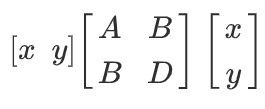# Homogeneous Polynomial

A homogeneous polynomial is a multivariate polynomial (i.e. a polynomial of more than one variable), with all terms of the same degree. Another way to put this: a polynomial is homogeneous of degree d if it is a linear combination of monomials of degree d .

General homogeneous polynomials are sometimes called polynomial forms:

• Degree 1 is a linear form,
• Degree 2 is a quadratic form,
• Degree 3 is a cubic form.

A homogeneous polynomial of degree k is also a homogeneous function of degree k. However, the converse is not true: there are many homogeneous functions that are not polynomials.

## Homogeneous Polynomial Examples

A more simple definition of a homogeneous polynomial is that that the sum of the exponents of the variables is the same for every term. For example, x3 + y3 = z3 or x2y3 = z5). The most famous example of a homogeneous polynomial is the Pythagorean theorem x2 + y2 = z2.

The expression x5 + 4x3y2 + 8xy4 is a homogeneous polynomial of degree 5. The sum of the exponents for each term gives you the degree of the term:

• x5 = 5
• 4x3y2 = 3 + 2 = 5
• 8xy4 = 8x1y4 = 4 + 1 = 5.

The product of two or more homogeneous polynomials is also a homogeneous polynomial, with a degree equal to the sum of the factor’s degrees. If all of the factors are not homogeneous, then the product is also not homogeneous. If the polynomial factors aren’t homogeneous, then neither is the product.

A homogeneous polynomial can be also be represented by a symmetric matrix. A symmetric matrix is a square matrix with symmetry around its leading diagonal, from top left to bottom right, as if you were folding along the diagonal. The top right half of the matrix and the bottom left half are mirror images about the diagonal. The following matrix represents Ax2 + 2Bxy + Dy2 :## References

 Bertram, A. Polynomials. Math 4800/6080 Project Course. Retrieved August 6, 2021 from: https://www.math.utah.edu/~bertram/4800/PolyIntroduction.pdf
 Blinn, J. (2003). Consider the Lowly 2 x 2 Matrix. In Jim Blinn’s Corner.

CITE THIS AS:
Stephanie Glen. "Homogeneous Polynomial" From StatisticsHowTo.com: Elementary Statistics for the rest of us! https://www.statisticshowto.com/homogeneous-polynomial/